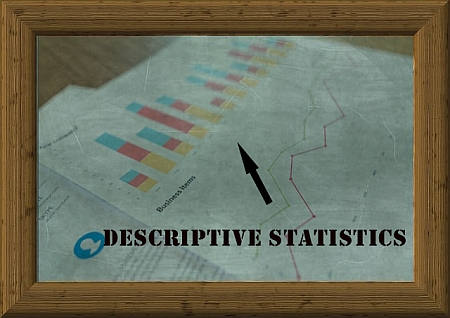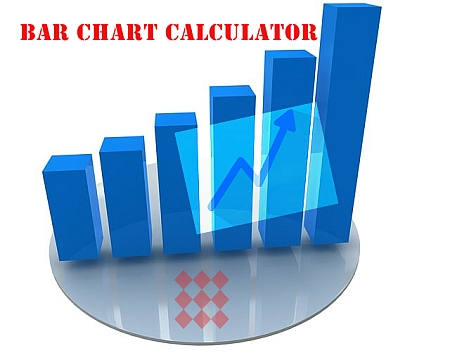# Descriptive Statistics Calculator

Instructions: Use this Descriptive Statistics Calculator to enter the sample data below and the solver will provide step-by-step calculation of the basic descriptive statistics, such as the mean, median, mode, variance, standard deviation, range, quartiles, 5-number summary, etc.Name of the variable (Optional)

## Descriptive Statistics Calculator

Descriptive Statistics corresponds to measures and charts that are derived from sample and are intended to provide information about the population being studied. Two basic types of descriptive statistics are the measures of central tendency and the measures of dispersion .### How do I compute descriptive statistics

The following are the typical steps you will follow to calculate descriptive statistics:

• Step 1: Identify clearly the sample data, and take note of the sample size n, which is the total number of data in the sample, included repeated values
• Step 2: Often times, you will want to sort the data in ascending order. Though this is not required to compute the mean and standard deviation, you will need to do it to compute the median and quartiles
• Step 3: Build classes with the data if you want to build a histogram. See the histogram calculator to see in detail how to construct these classes
• Step 4: With a set of numerical descriptive statistics and a graphical depiction provided by a histogram, you are now in a position to make some conclusions about the distribution of the data

### What do you use descriptive statistics for?

The measures of central tendency intend to give an idea of the location of the distribution. Examples of central tendency measures are the sample mean $$\bar X$$, the median and the mode . Examples of measures of dispersion are the sample variance $$s^2$$, the standard deviation $$s$$, and the range among others.

The sample mean is the most typical measures of central tendency used, as is the standard deviation the most commonly reported measure of dispersion.

The only possible drawback of those is that they are very sensitive to outliers, which means that their value can change dramatically with one or two strong outliers, if undetected or corrected for.

### Other common measures of central tendency and dispersion

As we mentioned in the previous paragraphs, outliers and strongly skewed distribution can affect dramatically the value of the mean and standard deviation.

Alternatively, for highly skewed data you can use the median or the midrange as measures of central tendency, and the interquartile range as a measure of dispersion.

### Descriptive Statistics Using Graphs

The charts that usually presented in a descriptive statistics report are the histogram and box plot , which give a very clear picture of the distribution of the variable that is being sampled.

Different measures are more appropriate than others for certain cases. For example, certain measures like the mean are very sensitive to outliers, and therefore, when a sample has strong outliers or it is very skewed, the preferred measure of central tendency would be the median instead of the sample mean### Descriptive Statistics Typically Reported

Usually, different formats are used, depending on the context of the sample data. Often times, the 5-number summary is reported, which consists of the Minimum , the first quartile, the median, the third quartile and the Maximum .

### What if I have Grouped Data

Grouped data needs to be handled differently, using frequency tables. When having grouped data, especially the type of data where we know the frequency associated to a given range of data, we need to proceed differently using an approximation of a midpoint to represent a range of data.

In that case you would instead use this descriptive statistics calculator for grouped data .

### Descriptive Statistics with Tables and Graphs

Often times, the point estimate of crucial population parameters such as the mean and standard deviation are extremely useful and can tell you a lot of about the population you are analyzing.

But at the same time, it is really important to use visual tools. For example, you can use this frequency distribution table calculator to condense the sample data into groups, and see how the data is clustered.

Or you can formally can construct a histogram, so to get a good depiction of the distribution of the population where the sample data is drawn from.

### Measures of Central Tendency

By far the most commonly used measure of central tendency is the sample mean, but it is not always appropriate for it to be used.

When the distribution is not bell-shaped, or strongly skewed, you will find a more representative measure of central tendency by using the median, mode, or even measures like the geometric mean and the harmonic mean.